# Catch up on the latest AI articles# GAN Also In The Time-series

3 main points
✔️ A review of research on the application of GANs to time series data generation
✔️ Demonstrate useful results by solving the challenges unique to GAN itself and time series
✔️ Privacy protection is one of the key challenges in time series data generation

Written by Eoin Brophy, Zhengwei Wang, Qi She, Tomas Ward
(Submitted on 23 Jul 2021)

Subjects: Machine Learning (cs.LG); Artificial Intelligence (cs.AI)code:## first of all

This is a survey paper of research on GAN applications to time series problems by a group at Dublin City University, Bight Dance. At the same time, a classification method is proposed. As is well known, most time-series data are normal, and collecting a large amount of outlier data requires a large number of resources. Therefore, various generative models have been proposed, and it is natural to consider the application of GAN.

The first model to be considered as a generative model is autoencoder (AE) shown in Fig. 1.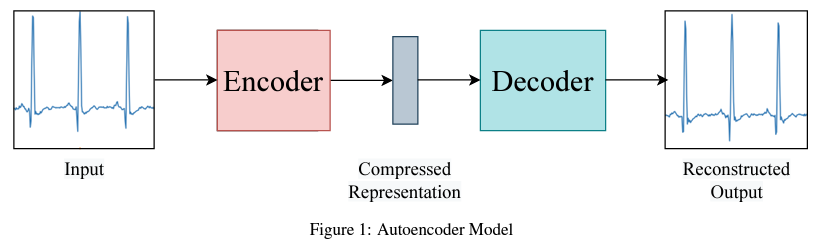Compared to AEs, GANs are front-runners due to the quality of the data they generate and their innate privacy protection.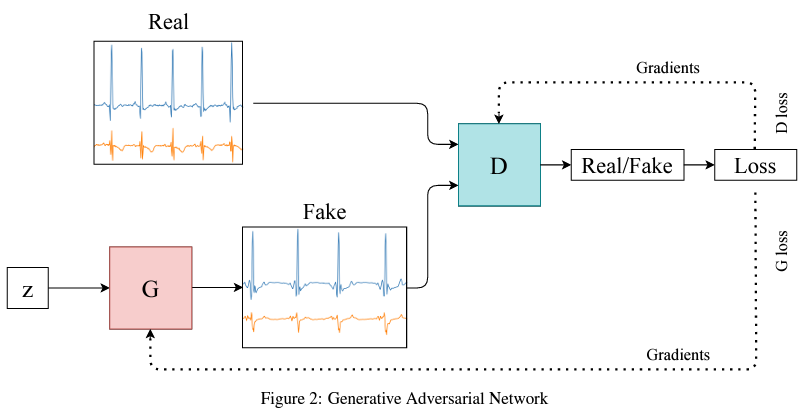The author presents review papers on GAN in other fields, which are omitted here.

## Challenges in applying to time series

The three main challenges are as follows

### Stability of learning

The stability of GANs, not only time series, has its challenges: two problems have been described in the original paper: 1) gradient vanishing and 2) mode collapse. The gradient vanishing is caused by the direct optimization of the loss function expressed by equation (1). When the discriminator D reaches its optimal state, optimizing (1) for the generator G minimizes the Jensen^Shannon (JS) divergence.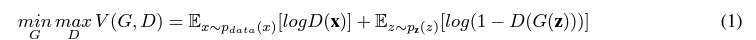JS convergence becomes a constant when there is no overlap in pr, pg. This results in a zero gradient. In practical use, there is a high probability that pr and pg do not intersect, or that the overlap is negligible.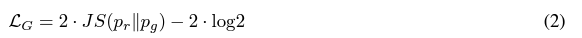To avoid this, the minimization in (3) is used to update the generator G.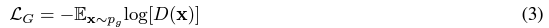This avoids the gradient vanishing but leads to the problem of mode collapse. The optimization of Eq. (3) is based on the inverse Kullback-Leibler (KL) divergence KL(pg ||p r ), which can be converted to When optimizing the inverse KL divergence if pr has multiple modes, pg will choose to recover one mode and ignore the others. Taking this into account, using (3) to training the generator G, can only generate a few modes of the actual data. These problems can be corrected by changing the architecture and the loss function.

### assess

Performance evaluation of GANs has been proposed extensively. The evaluation of GANs in computer vision is usually designed considering two aspects: the quality and quantity of the generated data. The most typical qualitative measure is the quality of the generated images, which is determined by human notation. Quantitative measures compare the generated image with the true image in terms of statistical properties, such as Maximum mean discrepancy (MMD ), Inception Score, and Frechet Inception Distance (FID ).

Compared to the evaluation of image-based GANs, time-series data is difficult to evaluate quantitatively in the human perceptual psychological sense. For qualitative evaluation, we usually use t-SNE or PCA to visualize how similar the two samples are. For quantitative evaluation, we apply the two-sample test as well as the image-based GAN.

### privacy risk

A wide range of methods is used to assess the privacy risks associated with the data generated by GANs.

## Commonly Used Data Sets

There is no standard or commonly used benchmarking datasets for time series data generation, such as image-based datasets (CIFAR, MNIST, ImageNet). table 1 shows a list of commonly used datasets. There are two repositories: the UCR Time Series Classification/Clustering database and the UCI Machine Learning repository.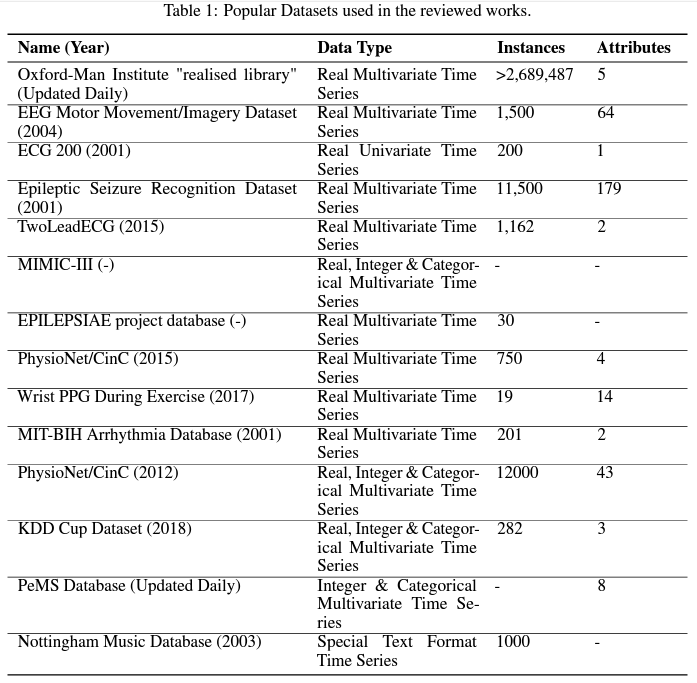## A Taxonomy of Time Series Based GANs

The data are classified as discrete and continuous variables. In discrete variables, data reporting is infrequent and irregular. Also, there are missing value gaps due to interruptions in reporting. Discrete-time series generation produces a sequence that may have temporal dependencies but may contain discrete signs. A continuous-time series has data corresponding to all-time points; Fig. 3 shows an example of each.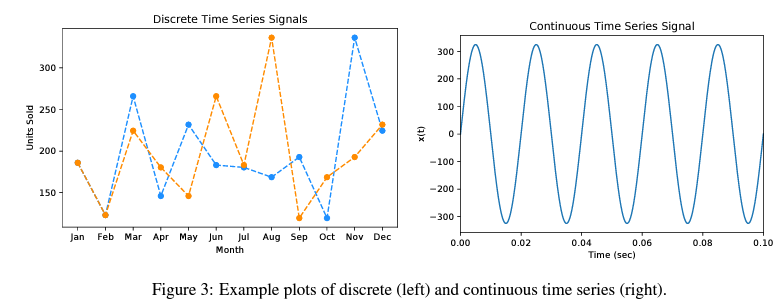### The challenge of generating discrete time series data

The obstacle to GANs is that they are zero-gradient at almost any point in time. This means that the distribution of discrete objects is not differentiable concerning their parameters. This limitation means that the generator cannot be trained simply by using backpropagation.

### The challenge of generating continuous time-series data

GAN originally deals with continuous data in the form of images. However, time series have another problem due to the time characteristic of continuous data. There is a complex correlation between temporal characteristics and their attributes. For example, when dealing with multi-channel biometric/physiological data, ECG characteristics depend on the age and health status of the individual. There are long-term correlations in time series data. Determining their length is more difficult than for image data. Transforming the dimensions of an image is a recognizable process, although it may result in a decrease in image quality. However, for continuous-time series data, there is no standard dimension (length) that can be used for GAN. This makes the benchmark comparison difficult.

RNN (Fig. 4) is suitable for handling sequential data because of its loop-like structure. However, it lacks the ability to learn long-term dependencies, so a variant, LSTM (Fig. 4, right), has been developed. Most of the papers discussed in this paper that have RNN-based architectures use LSTMs.

RNN-based Recurrent GAN (RGAN ) was proposed in 2016. It includes a recursive feedback loop in the generator.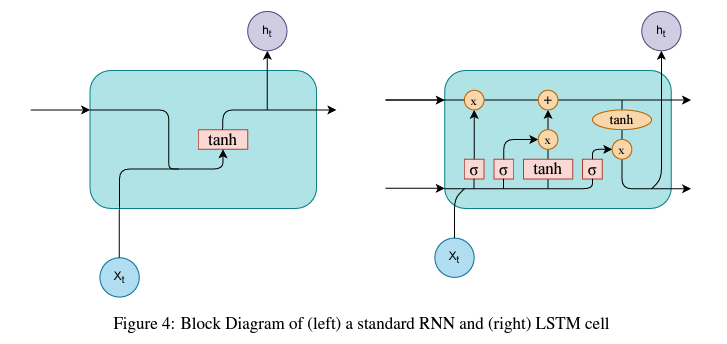### discrete variable GAN

・Sequence GAN (SeqGAN ) (Sept. 2016)

The SeqGAN generator contains LSTM cells and the discriminator is a Convolutional Neural Network (CNN), addressing the aforementioned challenges for discrete data, showing performance that outperforms other methods proposed up to 2016. The generator is updated by the measured gradient and the reward expectation from the discriminator with the Monte Carlo search. It looks like reinforcement learning. It was originally developed for discrete sequential data such as text but has opened the door to continuous sequential data and time series. The authors use synthetic data with a distribution generated by initializing the LSTM with random data that follows a normal distribution. They also compare the results with real-world data.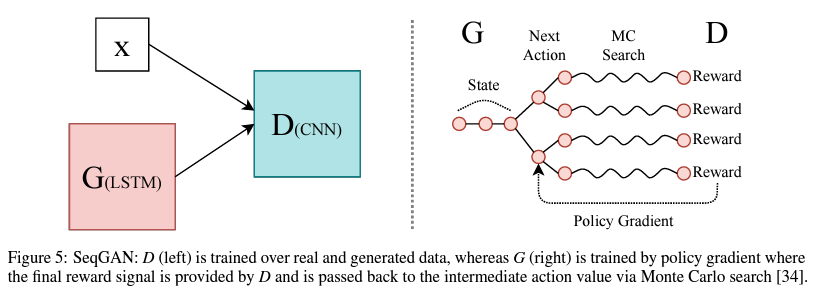・Quant GAN (Jul. 2019)

Quant GAN is a data-driven model that aims to capture the long-term dependence of financial time series data. Both the generator and the discriminator use a Temporal Convolutional Network (TCN) with skip connections, a Dilated Causal Convolutional Network like WaveNet. It is suitable for modeling the long-term range dependence of continuous sequential data. The function of the generator is a stochastic volatility neural network consisting of volatility and drift TCN; the time block used in the TCN consists of two Dilated Causal Convolution layers and two parametric ReLU activity functions. The data generated by the generator is passed to the discriminator for output, and the average value becomes the Monte Carlo prediction of the discriminator's loss function. Although the performance of the method outperforms conventional methods, the computational complexity of modeling long-term continuous time series data is a problem. Therefore, it was decided to apply the method to discrete data.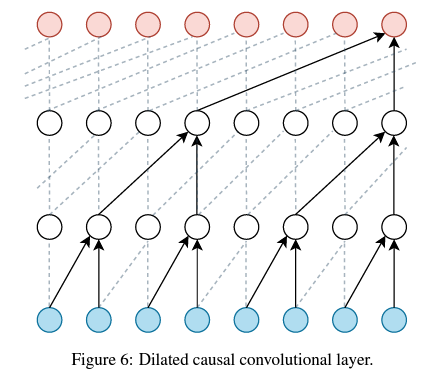### Continuous variable GAN

・Continuous RNN-GAN ( C-RNN-GAN ) (Nov.2016)

C-RNN-GAN generates continuous sequential data. The generator is an RNN and the discriminator is a bidirectional RNN. The RNN here is a two-stage LSTM.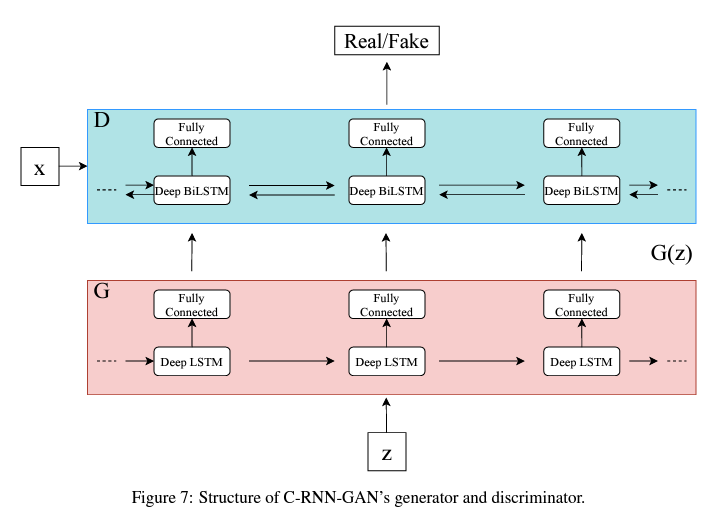・Recurrent Conditional GAN (RCGAN ) (2017)

The architecture of RCGAN is different from that of C-RNN-GAN; it uses the RNN LSTM, but the discriminator is not bidirectional. It uses RNN LSTMs, but the discriminator is not bi-directional, and the output of the generator is not fed back as input for the next time point. In this model, we input condition c and assign relevant labels to the time series data.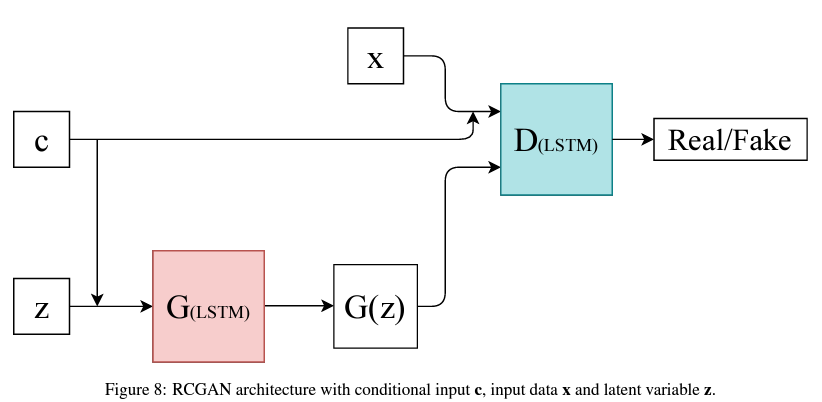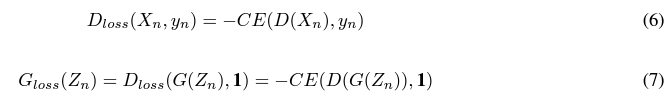Sequentially Coupled GAN (SC-GAN ) (Apr. 2019)

SC-GAN aims to generate patient-centric medical data that informs about the patient's current status and recommends medication dosages according to the patient's condition. 2 combined generators output the patient's current status and the recommended dosage, respectively. The discriminator is a two-layer bidirectional LSTM and both generators are two-layer unidirectional LSTMs. The generators are supervised and pre-trained.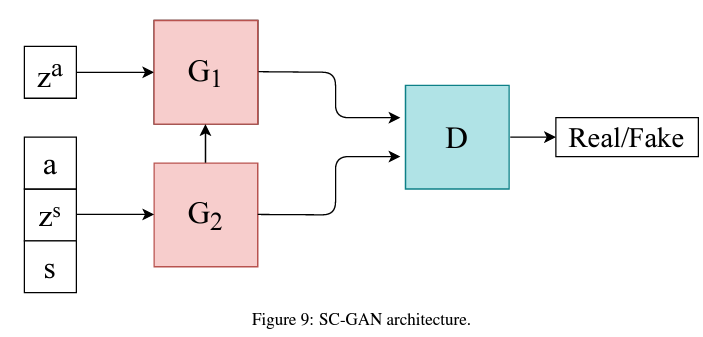Noise Reduction GAN (NR-GAN ) (Oct. 2019)

NR-GAN is intended for noise reduction of time series data, especially for EEG (electroencephalogram) signals. In particular, it is applied to denoise EEG (electroencephalogram) signals. nr-GAN removes noise in the frequency domain. The generator is a two-layer 1-D CNN with an all-coupled output layer, and the discriminator is a softmax layer instead of an all-coupled layer to compute probabilities. The generator does not sample from the latent space and tries to generate clear data from the raw EEG data. It is equivalent to a classical frequency filter but is limited by the amount of noise.